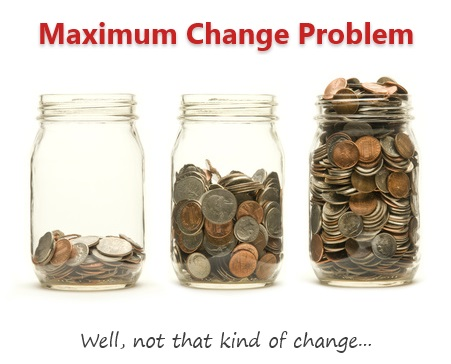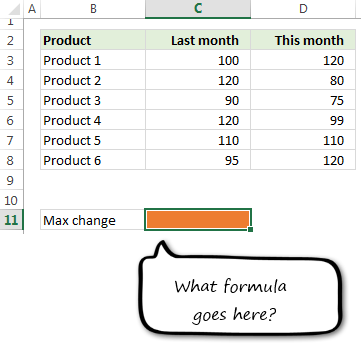# Calculating Maximum Change [solutions & discussion]

ShareLast Friday, we had a fun little Excel challenge – Calculate Maximum Change. More than 170 people commented and shared their solutions to this problem.

And the best part?

The best part is the variety of solutions & thinking displayed by our community. So if you are one of those 170, puff your chest & pat yourself on the back. Go ahead, I will wait.

Today, lets take a look at some of these awesome formulas and understand how they work. Read on and watch the video you below to gain few awesomeness pounds.

### First, lets understand the problem

Here is a look at the problem:• Are we talking about positive change, negative change or absolute change?
• Are we talking about % change or value change?

In the original problem, even though I did not mention it, most people assumed that we want absolute change of value (ie the answer is 40, for Product 2).

But in real life, you may want to understand the problem a little more before writing any formulas.

Note: The data is in C3:C8 for last month and D3:D8 for this month.

### Solution #1: Using MAX array formula

This is the solution most people got.

#### The array formula:

=MAX(C3:C8-D3:D8)

press CTRL+Shift+Enter after typing.

#### How it works?

C3:C8-D3:D8 portion:  This gives the result {-20;40;15;21;0;-25} in array form.MAX(…) portion: This simply calculates the maximum value of above array and returns 40 as answer.

Why press CTRL+Shift+Enter (CSE)?

We need to press CTRL+Shift+Enter because MAX() is not capable of handling arrays. If you write MAX({-20;40;15;21;0;-25}) you would get 40, but the same array when calculated by doing math on ranges will not work. To force MAX to treat arrays, we need to press CTRL+Shift+Enter.

### Solution #2: Using MAX+ABS array formula

Quite a few people figured out that the formula needs to work even when the change is negative. And that is where this new solution comes handy.

#### The array formula:

=MAX(ABS(C3:C8-D3:D8))

press CTRL+Shift+Enter after typing.

#### How it works?

ABS() portion: converts the change values {-20;40;15;21;0;-25} to positive {20;40;15;21;0;25}

Rest of the formula is same as solution #1.

### Solution #3: Using INDEX to avoid Ctrl+Shift+Enter

The thing with Ctrl+Shift+Enter is that you have to remember it. If you accidentally press Enter instead of CSE, the formula stops working. One way to avoid this is to route the calculation thru an Excel function that can natively process arrays. This is where INDEX (or SUMPRODUCT etc.) come handy.

The formula:

=MAX(INDEX(C3:C8-D3:D8,0))

or

=MAX(INDEX(ABS(C3:C8-D3:D8),0))

How it works?

Same as Solution #1, except for this formula you do not have to press Ctrl+Shift+Enter. The INDEX will automatically calculate the array and send numbers to MAX. Then MAX feels mighty comfortable dealing with those numbers and spits out the answer as 40.

### Solution #4: Using AGGREGATE

AGGREGATE() is a new function introduced in Excel 2010. This too, like INDEX & SUMPRODUCT can process arrays natively (provided you are using one of the aggregates like LARGE). Kyle, one of our commenters shared 2 brilliant solutions that involve AGGREGATE.

#### The formula:

=AGGREGATE(14,4,(C3:C8)-(D3:D8),1)

#### How it works?

14, 4 portion: This tells AGGREGATE that you want to calculate LARGE value (14) and you want to consider all cells (4). To understand more about AGGREGATE see the links below.

(C3:C8)-(D3:D8) portion: As seen above, this just gives an array – {-20;40;15;21;0;-25}

1 portion: This tells AGGREGATE that you want 1st largest number.

### Solution #5: Using MMULT and AGGREGATE

Now this is what I call a scary formula. It can potentially waste your entire afternoon when you try to understand it first time. But once you get it, you feel awesome. This too is posted by Kyle.

#### The formula:

=AGGREGATE(14,4,MMULT(C3:D8,{1;-1}),1)

#### How it works?

Watch the video. Explaining how this works in text is difficult.

I am still trying to understand MMULT(). It can be as complex and deep as string theory (or recipe of making bread at home). Go thru below links to learn more about it. Make sure you put on your helmet, cause it will blow your mind.

### More ways to get maximum change + Bonus problem

Watch below video to understand how to solve the maximum change problem and another related problem.

### What did you learn from this formula challenge?

I learned how to use AGGREGATE, Array SUMIFS and got a better handle on MMULT.

What about you? What did you learn thru this challenge. Please comment and let us all know.

### Get FREE Excel + Power BI Tips

Simple, fun and useful emails, once per week.

Learn & be awesome.

### Welcome to Chandoo.org

Thank you so much for visiting. My aim is to make you awesome in Excel & Power BI. I do this by sharing videos, tips, examples and downloads on this website. There are more than 1,000 pages with all things Excel, Power BI, Dashboards & VBA here. Go ahead and spend few minutes to be AWESOME.

Read my storyFREE Excel tips book

Chandoo is an awesome teacher
5/5

– Jason

Still on fence about Power BI? In this getting started guide, learn what is Power BI, how to get it and how to create your first report from scratch.

## Related Tips

### 11 Responses to “Calculating Maximum Change [solutions & discussion]”

1.Don says:

I disagree with all of the answers in your workbook. In the real world a product manager would want to know if the largest change was a negative or positive change. What good is it for a manager to know the absolute change when making decisions as to which product he/she wants to keep in stock or produce more. If answer is -40 not 40.

•Haz says:

This formula gives the real number instead of the absolute change:

{=INDEX(C3:C8-D3:D8-C3:C8,MATCH(MAX(ABS(C3:C8-D3:D8)),ABS(C3:C8-D3:D8),0))}

•Don says:

When I tried your formula I get -80. What am I missing.

•Don says:

My original posts:

{=IF(ABS(MIN(D3:D8-C3:C8))>MAX(D3:D8-C3:C8),MIN(D3:D8-C3:C8),MAX(D3:D8-C3:C8))}

And For Product:
{=INDEX(\$B\$3:\$B\$8,MATCH(IF(ABS(MIN(D3:D8-C3:C8))>MAX(D3:D8-C3:C8),MIN(D3:D8-C3:C8),MAX(D3:D8-C3:C8)),D3:D8-C3:C8,0))}

2.Jeff says:

This is a nice introduction to the ctrl+shift+enter for arrays. They are something I am aware of but rarely get around to applying. The combination of max and abs might be useful for me in analysing forecasts where I am interested in absolute variations over a period. The aggregate and index will be a topic for another rainy day.

3.Ritzie says:

Excellent post as always Chandoo ! Your website is what got me hooked to using excel more and more in the first place...and now every data problem I see, I itch to open my excel sheet for solving it 😛 Its a holy grail for excel enthusiasts 😀 Thankyou for being so awesome !!

A question on the above solution. How do we work the formula when it is max percentage change we are looking to find?? I tried some permutations combinations but dsnt seem to be working 🙁

4.Ville says:

Awesome! I also tried few things. I have to agree with DON about knowing if the value is negative or positive. Also one point would be knowing that what is the product that gives the difference.

So i placed in C11 =IF(ABS(MAX(INDEX(\$C\$3:\$C\$8-\$D\$3:\$D\$8;0)))>ABS(MIN(INDEX(\$C\$3:\$C\$8-\$D\$3:\$D\$8;0)));MAX(INDEX(\$C\$3:\$C\$8-\$D\$3:\$D\$8;0));MIN(INDEX(\$C\$3:\$C\$8-\$D\$3:\$D\$8;0)))

And in C12
=INDEX(\$B\$3:\$D\$8;MATCH(IF(ABS(MAX(INDEX(\$C\$3:\$C\$8-\$D\$3:\$D\$8;0)))>ABS(MIN(INDEX(\$C\$3:\$C\$8-\$D\$3:\$D\$8;0)));MAX(INDEX(\$C\$3:\$C\$8-\$D\$3:\$D\$8;0));MIN(INDEX(\$C\$3:\$C\$8-\$D\$3:\$D\$8;0)));INDEX(\$C\$3:\$C\$8-\$D\$3:\$D\$8;0);0);1)

This would find the maximum change in C11 and give the product that has the maximum change in C12.

For the percentile I would use (the percentile calculations in D11 & D12, format of D11 percent)
D11
=IF(ABS(MAX(INDEX((1-(\$C\$3:\$C\$8/\$D\$3:\$D\$8));0)))>ABS(MIN(INDEX((1-(\$C\$3:\$C\$8/\$D\$3:\$D\$8));0)));MAX(INDEX((1-(\$C\$3:\$C\$8/\$D\$3:\$D\$8));0));MIN(INDEX((1-(\$C\$3:\$C\$8/\$D\$3:\$D\$8));0)))

D12
=INDEX(\$B\$3:\$D\$8;MATCH(IF(ABS(MAX(INDEX((1-(\$C\$3:\$C\$8/\$D\$3:\$D\$8));0)))>ABS(MIN(INDEX((1-(\$C\$3:\$C\$8/\$D\$3:\$D\$8));0)));MAX(INDEX((1-(\$C\$3:\$C\$8/\$D\$3:\$D\$8));0));MIN(INDEX((1-(\$C\$3:\$C\$8/\$D\$3:\$D\$8));0)));INDEX((1-(\$C\$3:\$C\$8/\$D\$3:\$D\$8));0);0);1)

Wow, that was longer than expected. 🙂

What do you think?

•Ville says:

Uups. I had my columns mixed. I had this month in column C and last in column D. Also the percentile calculations are wrong.

Here are the corrected formulas:
C11
=IF(ABS(MAX(INDEX(\$D\$3:\$D\$8-\$C\$3:\$C\$8;0)))>ABS(MIN(INDEX(\$D\$3:\$D\$8-\$C\$3:\$C\$8;0)));MAX(INDEX(\$D\$3:\$D\$8-\$C\$3:\$C\$8;0));MIN(INDEX(\$D\$3:\$D\$8-\$C\$3:\$C\$8;0)))

C12
=INDEX(\$B\$3:\$D\$8;MATCH(IF(ABS(MAX(INDEX(\$D\$3:\$D\$8-\$C\$3:\$C\$8;0)))>ABS(MIN(INDEX(\$D\$3:\$D\$8-\$C\$3:\$C\$8;0)));MAX(INDEX(\$D\$3:\$D\$8-\$C\$3:\$C\$8;0));MIN(INDEX(\$D\$3:\$D\$8-\$C\$3:\$C\$8;0)));INDEX(\$D\$3:\$D\$8-\$C\$3:\$C\$8;0);0);1)

D11
=IF(ABS(MAX(INDEX(((\$D\$3:\$D\$8/\$C\$3:\$C\$8)-1);0)))>ABS(MIN(INDEX(((\$D\$3:\$D\$8/\$C\$3:\$C\$8)-1);0)));MAX(INDEX(((\$D\$3:\$D\$8/\$C\$3:\$C\$8)-1);0));MIN(INDEX(((\$D\$3:\$D\$8/\$C\$3:\$C\$8)-1);0)))

D12
=INDEX(\$B\$3:\$D\$8;MATCH(IF(ABS(MAX(INDEX(((\$D\$3:\$D\$8/\$C\$3:\$C\$8)-1);0)))>ABS(MIN(INDEX(((\$D\$3:\$D\$8/\$C\$3:\$C\$8)-1);0)));MAX(INDEX(((\$D\$3:\$D\$8/\$C\$3:\$C\$8)-1);0));MIN(INDEX(((\$D\$3:\$D\$8/\$C\$3:\$C\$8)-1);0)));INDEX(((\$D\$3:\$D\$8/\$C\$3:\$C\$8)-1);0);0);1)

I hope they are correct this time around. 🙂

What do you think?

5. […] challenged us to solve an Excel problem, and find the products with the maximum change in monthly sales. There were 170 comments, with a wide variety of […]

6.Adi says:

Hi Chandoo,

One more simple formula: 'Large' which is array entered.
i.e. =large(ABS(C3:C8-D3:D8),1) array entered.

### Get FREE Excel & Power-BI Newsletter

One email per week with Excel and Power BI goodness. Join 100,000+ others and get it free.